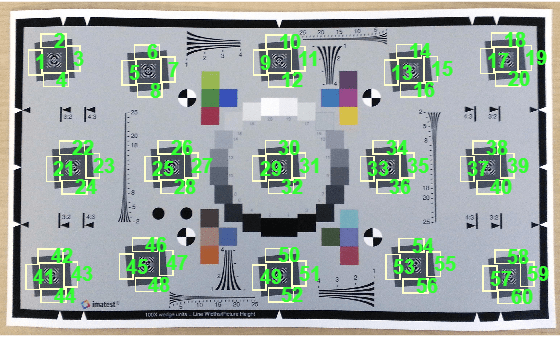# measureChromaticAberration

Measure chromatic aberration at slanted edges using Imatest eSFR chart

## Syntax

``aberrationTable = measureChromaticAberration(chart)``
``aberrationTable = measureChromaticAberration(chart,Name,Value)``

## Description

example

````aberrationTable = measureChromaticAberration(chart)` measures the chromatic aberration at all slanted edge regions of interest (ROIs) of an Imatest® Extended eSFR chart .```
````aberrationTable = measureChromaticAberration(chart,Name,Value)` measures the chromatic aberration with additional parameters to specify a subset of ROIs to measure.```

## Examples

collapse all

Read an image of an eSFR chart into the workspace.

`I = imread('eSFRTestImage.jpg');`

Create an `esfrChart` object, then display the chart with ROI annotations. The 60 slanted edge ROIs are labeled with green numbers.

```chart = esfrChart(I); displayChart(chart,'displayColorROIs',false,... 'displayGrayROIs',false,'displayRegistrationPoints',false)```Measure the chromatic aberration in all slanted edge ROIs. Examine the contents of the returned table, `chTable`, for a single ROI.

```chTable = measureChromaticAberration(chart); ROIIndex = 3; chTable(3,:)```
```ans=1×5 table ROI aberration percentAberration edgeProfile normalizedEdgeProfile ___ __________ _________________ _____________ _____________________ 3 1.9164 0.14883 {348x4 table} {348x4 table} ```

Store the normalized edge profile in a separate variable, `edgeProfile`, for clarity. Examine the normalized color intensity of the first and last pixel of `edgeProfile`.

```edgeProfile = chTable.normalizedEdgeProfile{ROIIndex}; edgeProfile([1 end],:)```
```ans=2×4 table normalizedEdgeProfile_R normalizedEdgeProfile_G normalizedEdgeProfile_B normalizedEdgeProfile_Y _______________________ _______________________ _______________________ _______________________ -0.0022049 0.0094112 0.0088172 0.0050968 0.98511 0.98953 1.01 0.986 ```

Plot the normalized intensity for the ROI.

```npix = length(edgeProfile.normalizedEdgeProfile_R); plot(1:npix,edgeProfile.normalizedEdgeProfile_R,'r', ... 1:npix,edgeProfile.normalizedEdgeProfile_G,'g', ... 1:npix,edgeProfile.normalizedEdgeProfile_B,'b') xlabel('Pixel') ylabel('Normalized Intensity') title(['ROI ' num2str(ROIIndex) ' with Aberration ' num2str(chTable.aberration(ROIIndex))])```The blue channel has a higher intensity than the red and green channels immediately before the edge, and a lower intensity than the red and green channels immediately after the edge. This difference in intensity contributes to the measured value of chromatic aberration.

The measured values of `aberration` and `percentAberration` for this edge are relatively small. Visual inspection of the image confirms that the sides of the edge do not have a strong color tint.

## Input Arguments

collapse all

eSFR chart, specified as an `esfrChart` object.

### Name-Value Pair Arguments

Specify optional comma-separated pairs of `Name,Value` arguments. `Name` is the argument name and `Value` is the corresponding value. `Name` must appear inside quotes. You can specify several name and value pair arguments in any order as `Name1,Value1,...,NameN,ValueN`.

Example: `measureChromaticAberration(myChart,'ROIIndex',2)` measures the chromatic aberration only of ROI 2.

ROI indices to include in measurements, specified as the comma-separated pair consisting of `'ROIIndex'` and a scalar or vector of integers in the range [1, 60]. The indices match the ROI numbers displayed by `displayChart`.

### Note

`measureChromaticAberration` uses the intersection of ROIs specified by `'ROIIndex'` and `'``ROIOrientation``'`.

Example: `29:32`

Data Types: `single` | `double` | `int8` | `int16` | `int32` | `uint8` | `uint16` | `uint32`

ROI orientation, specified as the comma-separated pair consisting of `'ROIOrientation'` and `'both'`, `'vertical'`, or `'horizontal'`. The `measureChromaticAberration` function performs measurements only on ROIs with the specified orientation.

### Note

`measureChromaticAberration` uses the intersection of ROIs specified by `'``ROIIndex``'` and `'ROIOrientation'`.

Example: `'vertical'`

Data Types: `char` | `string`

## Output Arguments

collapse all

Chromatic aberration measurements, returned as an m-by-5 table. m is the number of sampled ROIs.

The five columns represent these variables:

VariableDescription
`ROI`Index of the sampled ROI. The value of `ROI` is an integer in the range [1, 60].
`aberration`

Chromatic aberration, measured as the area between the maximum and the minimum red, green, and blue edge intensity profiles. The measured chromatic aberration indicates perceptual chromatic aberration. `aberration` is a scalar of type `double`.

`percentAberration`Aberration, expressed as a percentage of the distance in pixels between the center of the image and the center of the ROI.
`edgeProfile`

Intensity profile of each color channel across the edge in the ROI. `edgeProfile` is an s-by-4 table, where s is the number of samples across the edge. The four columns represent the red, green, blue, and luminance values, averaged along the edge.

Luminance (Y) is a linear combination of the red (R), green (G), and blue (B) channels according to:

Y = 0.213R + 0.715G + 0.072B

### Note

The sampling rate for the chromatic aberration measurement is about four times the sampling rate of the image.

`normalizedEdgeProfile`

Intensity profile, normalized between [0, 1] using 5% of the front end and tail end of data. `normalizedEdgeProfile` is an s-by-4 table with a similar structure to `edgeProfile`.

## Tips

• Chromatic aberration is best measured at slanted edges that are:

• Roughly orthogonal to the line connecting the center of the image and the center of the ROI

• Farthest from the center of the image

Because chromatic aberration increases radially from the center of the image, measurements at slanted edges near the center of the image can be ignored.

• The absolute chromatic aberration reported in the `aberration` field is measured in the horizontal or vertical direction. However, chromatic aberration is a radial phenomenon, and radial measurements are more accurate.